Name:    3rd Grade Math Proficient Test 11

Multiple Choice
Identify the choice that best completes the statement or answers the question.

1.

Statistics/Probability and Graphing – Combinations & Permutations – RIT191 – 200

In how many different ways can the letters in the word MATH be
arranged?
 a. 4 d. 16 b. 8 e. 24 c. 12

2.

Statistics/Probability and Graphing – Graphing – RIT 191 – 200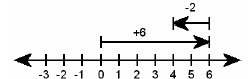What subtraction sentence is modeled on the number line shown above?
 a. 3 + 6 = 9 d. 6 – 4 = 2 b. 6 – 2 = 4 e. 4 – 2 = 2 c. 2 + 6 = 8

3.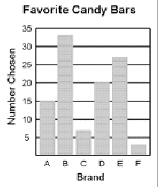How many people choose brand E?
 a. 7 d. 27 b. 20 e. 33 c. 15

4.

Look at the graph. It represents the number of milkshakes ordered at
“Shakeland” in a three hour period.a. 15 d. 5 b. 10 e. 2 c. 8

5.

The line graph shows the number of students that were absent from a third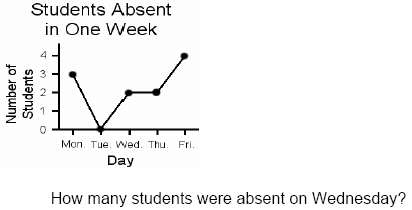a. 0 d. 3 b. 1 e. 4 c. 2

6.a. blue d. hazel b. brown e. other c. green

7.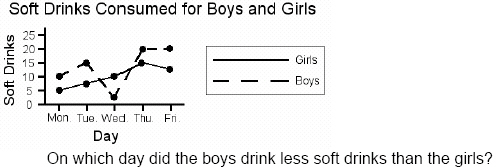a. Monday d. Thursday b. Tuesday e. Friday c. Wednesday

8.

Statistics/Probability and Graphing – Probability and Prediction – RIT 191 –200

Without looking, you pull out only one M&M from a bag that contains 5 red, 10
green, 5 yellow, 5 orange, and 5 blue M&M’s. What color are you most
likely to pull out?
 a. red d. orange b. green e. blue c. yellow

9.

Statistics/Probability and Graphing – Statistics – RIT 191 – 200

In a survey of 30 seventh graders' favorite colors, 5 chose red, 10 chose blue,
7 chose green, and 8 chose yellow. What is the correct tally entry for green?
 a.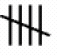b.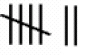c.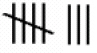d.e.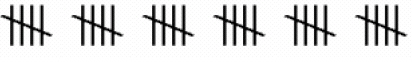10.

Statistics-Probability-Graphing191-200a. 7 d. 8 b. 2 e. 3 c. 4

11.a. More than half of the students chose math. b. The least number of students chose art. c. Half of the students chose reading. d. The greatest number of students chose science. e. Half of the students chose music.

12.

Statistics/Probability and Graphing – Probability and Prediction – RIT 201 –210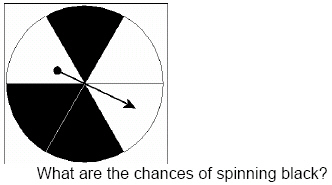a. one out of six d. five out of six b. three out of four e. three out of six c. one out of three

13.The spinner will:
 a. usually land on blue. d. always land on green. b. always land on blue. e. never land on blue. c. usually land on green.

14.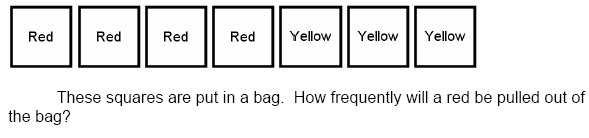a. 4 out of 7 d. 0 out of 7 b. 3 out of 7 e. 10 out of 7 c. 7 out of 7

15.

Statistics/Probability and Graphing – Statistics – RIT 201 – 210

Sal sold 20 pizzas on Monday, 17 on Tuesday, and 23 on Wednesday.
What is the average number of pizzas Sal sold?
 a. 60 d. 17 b. 30 e. 20 c. 23

16.

Statistics/Probability and Graphing – Graphing – RIT 211 – 220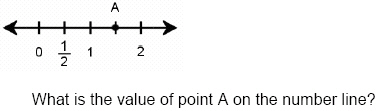a.d.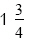b.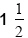e.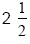c.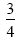17.a. –4, 4, -1, 3 d. –4, -3, 1, 2 b. –1, -4, 3, 4 e. –4, -1, 2, 3 c. 4, 3, -1, -4